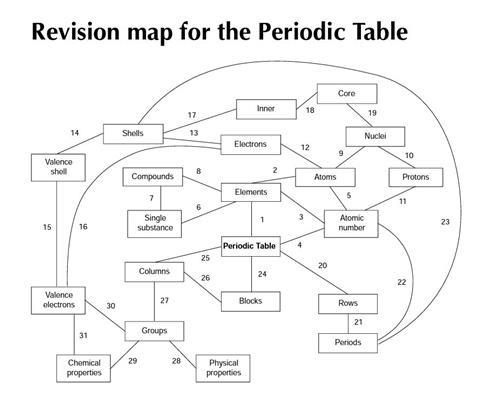The Periodic Table is one of the central organising themes in chemistry

It is one of the areas where ideas about the macroscopic (i.e. properties of elements) and molecular (e.g. electronic configuration) properties are related.

Students will be given a copy of a revision ‘map’ for the topic of the Periodic Table. The map is a diagram with boxes labelled with some words we use in chemistry, joined by numbered lines. Each of the lines suggests a relationship, which could be described in a sentence.

You are asked to think up sentences to show how the different words in the boxes are related. Try and be as accurate and precise as you can. Make sure you put each sentence next to the correct number.A shorter, simplified version of this resource is available as part of a complete lesson plan for 14–16 year olds on revising the periodic table using a concept map.

Here are some suggested sentences linking the words in the diagram.

1. The Periodic Table is a way of arranging what we know about the chemical elements.
2. Each element in the Periodic Table is a different type of atom.
3. Each element has a different atomic number.
4. The Periodic Table is arranged in atomic number order.
5. Each atom has an atomic number.
6. An element is a single chemical substance.
7. A compound is a single chemical substance.
8. Compounds are formed when two or more chemical elements react together.
9. An atom has a central nucleus.
10. A nucleus contains one or more protons.
11. The number of protons in the atomic nucleus gives the atomic number.
12. An atom contains one or more electrons.
13. The electrons are arranged in shells.
14. The outermost shell is called the valence shell.
15. Electrons in the valence shell are called valence electrons.
16. Each atom has one or more valence electrons.
17. Most atoms have one or more inner shells of electrons inside the valence shell.
18. The inner shells are part of the atomic core.
19. The nucleus is part of the atomic core.
20. The elements are arranged in rows in the Periodic Table.
21. The rows of elements are called periods.
22. The higher the atomic number of an element, the higher the period.
23. The number of shells of electrons determines the period an element is in.
24. The Periodic Table is arranged in several blocks.
25. The elements are arranged in columns in the Periodic Table.
26. Each block of the Periodic Table contains several columns that are next to each other.
27. The columns of elements are called groups.
28. Physical properties of elements often change in a pattern down the group.
29. Groups of elements usually have similar chemical properties.
30. Elements in a group have the same number of outer valence electrons.
31. An element’s chemical properties are related to the number of outer valence electrons in its atoms.